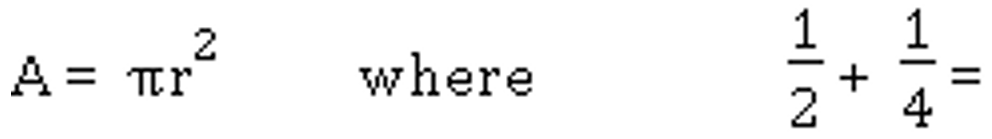•Fixing Data Interoperability

# EdSun.com

#### Educational Solutions Unified Network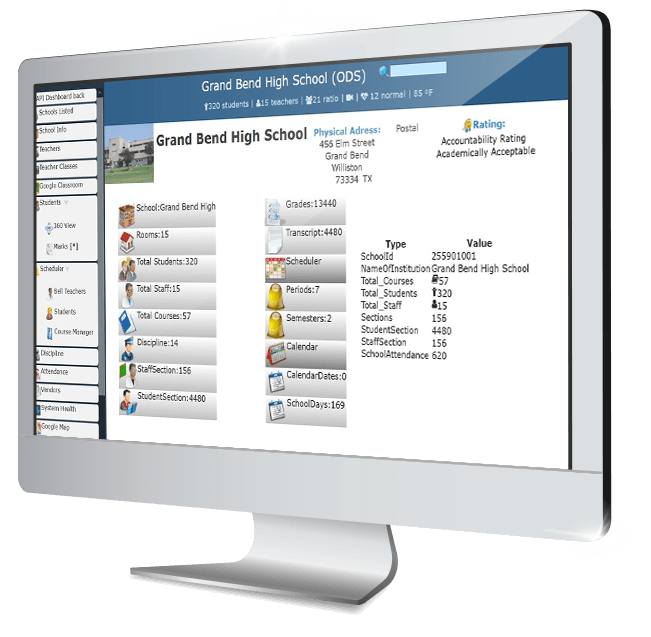• Sync EdFi data with Google classroom
• View Google Classroom from the Visual Dashboard•

### Optimize Education

• Student requests
• Class size
• Early warning
• Chronic absenteeism

With Data Interoperability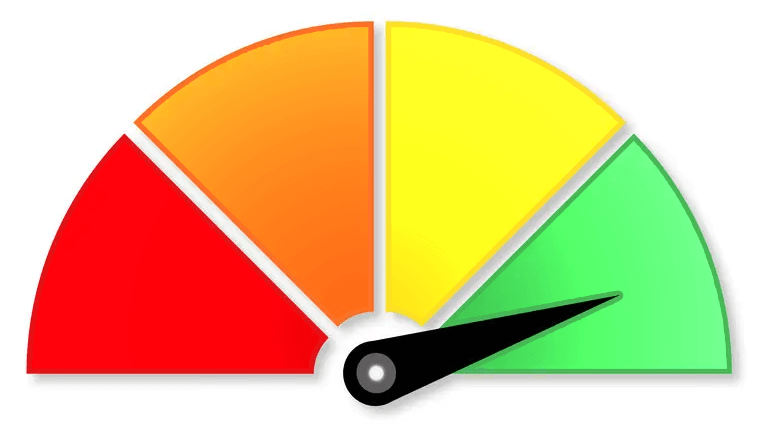• ### District Portal

• Insights
• Assessments
• Visual Dashboard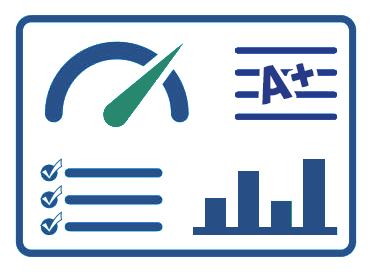• ### Mobile App

Mobile Data Interoperability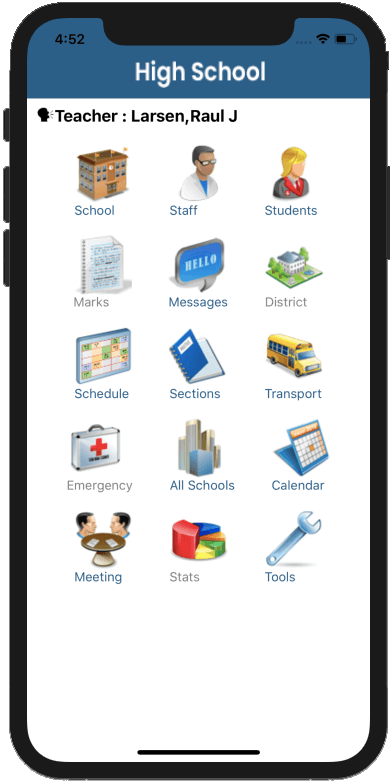• ### Fixing Data Interoperability

#### By integrating complex big data systems

Big data integration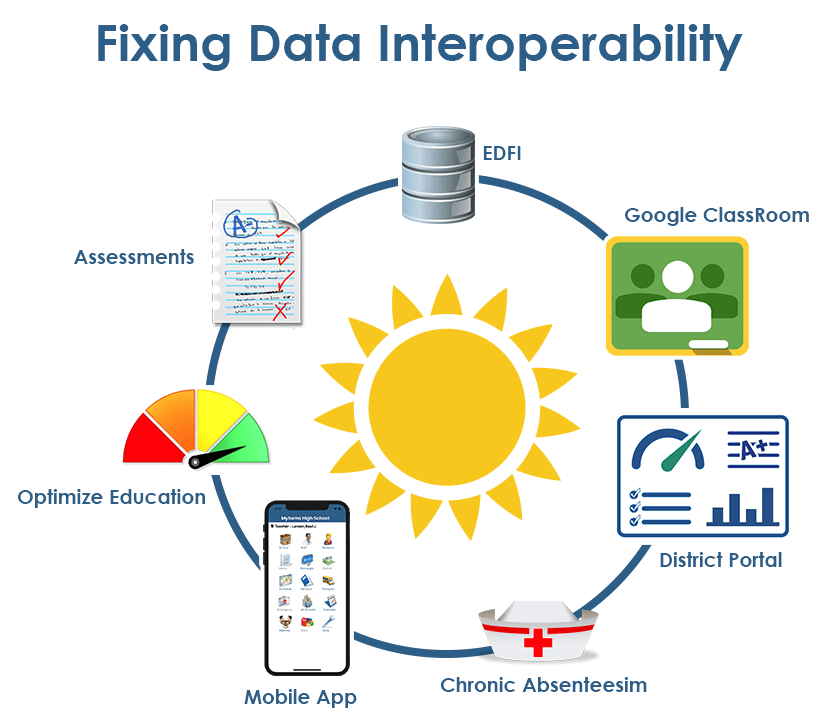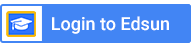## Online Instruction in Math### Edsun.com

Mathematics
Type: Online
Duration: 30 minutes
Assignment

Complete the following questions:

1) Numbers

(a) There are ____ rows with ____ apples in each row.
(b) We can count in multiples of ____
(c) Multiples of ____ are ____
(d) Write an addition number sentence: __________________
(e) Write a multiplication number sentence: __________________
(f) There are ____ columns with ____ circles in each column.
(g) We can count in multiples of __, _, _, _, _, _, _, _, _, _, _, _, _, _, _.
(h) Multiples of __________ are ___________
(i) Complete: There are _________________________ apples
(j) What color are the apples : __________________

2) Number meanings

What is 1+1=[__] * 3= [__] +5=[__]

The final answer above is : [even/odd] number

The final answer above is a : [prime/not prime] number

3) Number Missing

What is the missing number?

 1 8 5 7 ? 9 4 2 3

The missing number is:______

4) Formulas